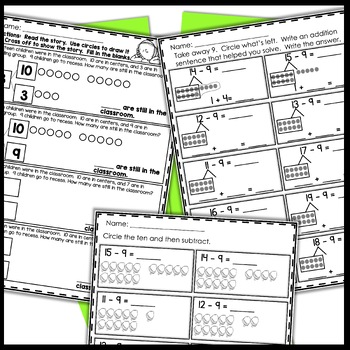# 1st Grade Math Worksheets |Make a Ten, Take From Ten| Independent Work PacketsSubject
Resource Type
File Type

Zip

(59 MB|74 pages)
Standards
Also included in:
1. These print and go NO PREP math worksheets are the perfect addition to your first grade curriculum. They cover all first grade concepts in an easy to read layout. These are perfect for reinforcement of skills taught, homework, independent work, or group work.YOU WILL SAVE 20% IF YOU PURCHASE THIS
\$63.50
\$47.62
Save \$15.88
• Product Description
• StandardsNEW

These Print and Go Math Sheets cover counting on, making ten to solve, taking 10 from a teen number, and total unknown. All you need to do is PRINT and GO! These are great as supplemental material, homework, reinforcement, or just plain FUN! Yep, I said it - FUN! I LOVE MATH! If you use Eureka Math these are great first grade math worksheets that can be used as supplemental activities. This can be used as Independent Work Packets.

BUNDLED FOR CONVENIENCE

★SCHOOL DISTRICTS★

★SCHOOL DISTRICTS TPT offers PO sales!  Here's the LINK to read all about it!  Thanks TPT for making this easy for schools.  If you need to contact me for a preview of one of my bundles, please email me at scjuneau@yahoo.com.

★EARN TPT DOLLARS FOR FUTURE PRODUCTS★

★CUSTOMER TIPS:How to get TPT credit (\$\$\$) to use on future purchases: • Please go to your My Purchases page (you may need to login). Beside each purchase you'll see a Provide Feedback button. Simply click it and you will be taken to a page where you can give a quick rating and leave a short comment for the product. I value your feedback greatly as it helps me determine which products are most valuable for your classroom so I can create more for you. Be the first to know about my new discounts, freebies and product launches: • Look for the STAR near the top of any page within my store and click it to become a follower. You will now receive customized email updates about my store.

★THANK YOU FOR CHECKING OUT MY LITTLE STORE!

I SURE APPRECIATE YOU!★

Understand the meaning of the equal sign, and determine if equations involving addition and subtraction are true or false. For example, which of the following equations are true and which are false? 6 = 6, 7 = 8 - 1, 5 + 2 = 2 + 5, 4 + 1 = 5 + 2.
Add and subtract within 20, demonstrating fluency for addition and subtraction within 10. Use strategies such as counting on; making ten (e.g., 8 + 6 = 8 + 2 + 4 = 10 + 4 = 14); decomposing a number leading to a ten (e.g., 13 - 4 = 13 - 3 - 1 = 10 - 1 = 9); using the relationship between addition and subtraction (e.g., knowing that 8 + 4 = 12, one knows 12 - 8 = 4); and creating equivalent but easier or known sums (e.g., adding 6 + 7 by creating the known equivalent 6 + 6 + 1 = 12 + 1 = 13).
Relate counting to addition and subtraction (e.g., by counting on 2 to add 2).
Understand subtraction as an unknown-addend problem. For example, subtract 10 – 8 by finding the number that makes 10 when added to 8.
Apply properties of operations as strategies to add and subtract. If 8 + 3 = 11 is known, then 3 + 8 = 11 is also known. (Commutative property of addition.) To add 2 + 6 + 4, the second two numbers can be added to make a ten, so 2 + 6 + 4 = 2 + 10 = 12. (Associative property of addition.)
Total Pages
74 pages
N/A
Teaching Duration
N/A
Report this Resource to TpT
Reported resources will be reviewed by our team. Report this resource to let us know if this resource violates TpT’s content guidelines.